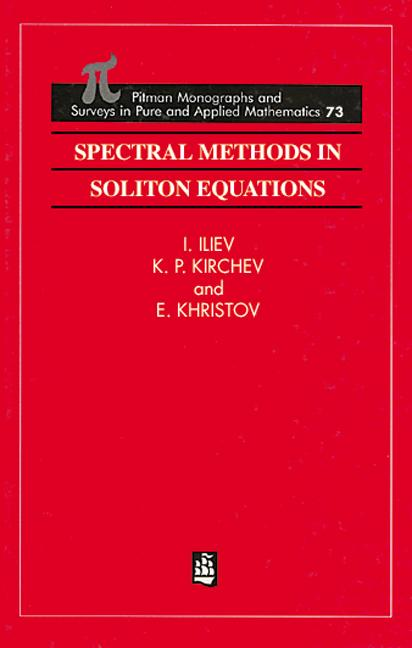# Spectral Methods in Soliton Equations

## 1st Edition

Chapman and Hall/CRC

400 pages

##### Purchasing Options:\$ = USD
Hardback: 9780582239630
pub: 1994-11-21
Currently out of stock
\$350.00
x

FREE Standard Shipping!

### Description

Soliton theory as a method for solving some classes of nonlinear evolution equations (soliton equations) is one of the most actively developing topics in mathematical physics. This book presents some spectral theory methods for the investigation of soliton equations ad the inverse scattering problems related to these equations. The authors give the theory of expansions for the Sturm-Liouville operator and the Dirac operator. On this basis, the spectral theory of recursion operators generating Korteweg-de Vries type equations is presented and the Ablowitz-Kaup-Newell-Segur scheme, through which the inverse scattering method could be understood as a Fourier-type transformation, is considered. Following these ideas, the authors investigate some of the questions related to inverse spectral problems, i.e. uniqueness theorems, construction of explicit solutions and approximative methods for solving inverse scattering problems. A rigorous investigation of the stability of soliton solutions including solitary waves for equations which do not allow integration within inverse scattering method is also presented.

Introduction

Spectral theory of the regular A-operators

Spectral theory for A-operators on the semi-axis

A-operators on the line and nonlinear evolution equations

Stability of solitary wave solutions for nonlinear evolution equations

Bibliography

### Subject Categories

##### BISAC Subject Codes/Headings:
MAT007000
MATHEMATICS / Differential Equations
SCI040000
SCIENCE / Mathematical Physics